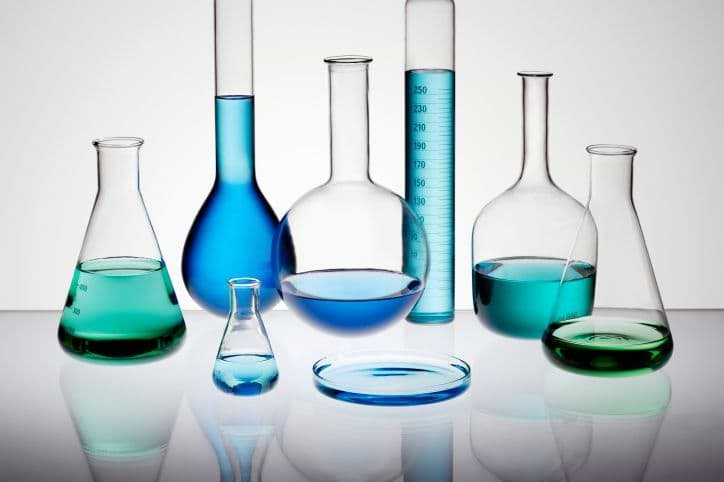Education

# What are the Gas Laws in Chemistry?Written by

The gas laws can be used to predict the behaviour of gases under certain physical conditions. Examples of gas laws include Charles’ law, Boyle’s law, and Gay-Lussac’s law. These laws provide relationships between:
The pressure exerted by gaseous substances on the walls of their containers (often denoted by ‘P’)
The absolute temperature associated with the gas (denoted by the symbol ‘T’ and expressed in Kelvin)
The number of moles of gas or the amount of gaseous substance (generally denoted by ‘n’)
The volume occupied by the gas (often denoted by the symbol ‘V’ and expressed in terms of litres)
A brief description of three of the gas laws is provided in this article.

Boyle’s Law

Boyle’s law states that the pressure exerted by a gas on its container increases as the volume of the container decreases (when the absolute temperature of the gas and the number of gaseous moles are constant).
The mathematical expression of this law is:
P = k/V (or) PV = k
Where P is the pressure associated with the gas, V is the volume of the gas, and k is a constant.

Charles’ Law

Charles’ law can be stated as follows – “When the number of gaseous moles and the pressure of the gas is kept constant, the volume occupied by a gaseous substance is directly proportional to the absolute temperature associated with the gas”.
This law can be expressed as follows:
V/T = k (or) V = kT
Where V is the volume occupied by the gas, T is the absolute temperature of the gas, and k is a constant.